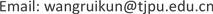1. 引言

Bharati  等人提出了一种新的使用有监督的深度玻尔兹曼机的算法，在人脸图片中选取了4个部分学习特征，通过使用提出的算法判别人脸图像是修饰过的还是原始图像。该方法并没有检测图像是否发生变形篡改以及定位篡改发生的位置。

Hwang  提出了一种图像局部变形的检测方法，他们通过研究几何变换中的差值模式，提出了一种重新插值的方法检测几何变形，但是这种需要直观观察的方法在图像局部几何变形中的检测效果并不是很理想。对于压缩图像的篡改的检测效果更是不好。图1显示了使用Hwang的方法检测图像局部集合变相的结果。

JPEG在压缩的过程中是对图像进行分块处理的，对每个压缩块计算其离散余弦变换(DCT)，这种压缩方法使得图像不同块之间的关系发生弱化，块中像素相关性较大而块间的像素相关性变弱，造成快件出现断层，即块效应。块效应常用来检测图像的复制粘贴及拼接操作，人们在对图像进行上述篡改操作时主要考虑将复制或剪切的部分放置在图像的合适位置，并不考虑图像的块效应是否一致。同样，在对图像进行局部变形时会改变变形区域像素的值，使像素发生移动的现象，这种情况破坏了压缩图像的块效应。图2展示了对于压缩未篡改图像的块效应网格以及压缩篡改图像的块效应网格。

2. 提出的方法

2.1. 缩放残差图像提取

I ( x , y ) → STEP1 I ′ ( x ′ , y ′ ) → STEP2 I ^ ( x ′ , y ′ ) (1)

step1 = { 0 ≤ x ≤ N x − 1 , x ∈ z 0 ≤ y ≤ N y − 1 , y ∈ z (2)

step2 = { 0 ≤ x ′ ≤ N x − 2 , x ∈ z 0 ≤ y ′ ≤ N y − 2 , y ∈ z (3)

K ( K ) = { ( a + 2 ) | x | 3 − ( a + 3 ) | x | 2 + 1 ,   for   | x | ≤ 1 a | x | 3 − 5 a | x | 2 + 8 a | x | − 4 a ,   for   1 < | x | < 2 0 ,   otherwise (4)

g ( x , y ) = ∑ l = − 1 2 ∑ m = − 1 2 C i + l , j + m K ( k x ) K ( k y ) (5)

D ( x , y ) = I ( x , y ) − I ^ ( x ′ , y ′ ) (6)

2.2. BAG提取

2.2.1. 提取弱水平线E<sub>h</sub>

e I ( x , y ) = ∑ i = y − 16 16 d ( x , i ) (7)

e ( x , y ) = e I ( x , y ) − M i d [ { e I ( i , y ) | x − 16 ≤ i ≤ y + 16 } ] (8)

2.2.2. 从图像E<sub>h</sub>中提取具有8周期的行

g h ( x , y ) = M i d [ { e ( i , y ) | i = x − 16 , x − 8 , x , x + 8 , x + 16 } ] (9)

g ( x , y ) = g h ( x , y ) + g v ( x , y ) (10)

2.2.3. 计算图像G的特征f

f = a v e [ ∑ i = 2 7 ∑ j = 2 7 a ( i , j ) ] − a v e [ { ∑ i = 1 8 a ( x , i ) | x = 1 , 8 } + { a ( x , 1 ) | 2 ≤ x ≤ 7 } + { a ( x , 8 ) | 2 ≤ x ≤ 7 } ] + b (11)

3. 实验分析

3.1. 特征偏移参数选择

3.2. 方法实验结果

4. 总结